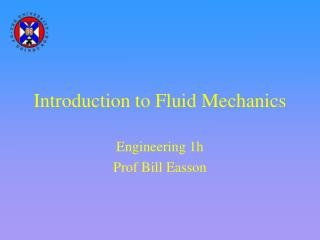# Introduction to Fluid Mechanics - PowerPoint PPT PresentationDownload PresentationIntroduction to Fluid Mechanics

Introduction to Fluid Mechanics
Download Presentation## Introduction to Fluid Mechanics

- - - - - - - - - - - - - - - - - - - - - - - - - - - E N D - - - - - - - - - - - - - - - - - - - - - - - - - - -
##### Presentation Transcript

1. Introduction to Fluid Mechanics Engineering 1h Prof Bill Easson

2. Definition of a Fluid A fluid is a substance that flows under the action of shearing forces. If a fluid is at rest, we know that the forces on it are in balance. A gas is a fluid that is easily compressed. It fills any vessel in which it is contained. A liquid is a fluid which is hard to compress. A given mass of liquid will occupy a fixed volume, irrespective of the size of the container. A free surface is formed as a boundary between a liquid and a gas above it.

3. Density The density of a fluid is defined as its mass per unit volume. It is denoted by the Greek symbol, . kg  water= 998 kgm-3 m  = V air =1.2kgm-3 kgm-3 m3 If the density is constant (most liquids), the flow is incompressible. If the density varies significantly (eg some gas flows), the flow is compressible. (Although gases are easy to compress, the flow may be treated as incompressible if there are no large pressure fluctuations)

4. Pressure Pressure is the force per unit area, where the force is perpendicular to the area. N pa= 105 Nm-2 F p= Nm-2 (Pa) A 1psi =6895Pa m2 This is the Absolute pressure, the pressure compared to a vacuum. The pressure measured in your tyres is the gauge pressure, p-pa.

5. Pressure Pressure in a fluid acts equally in all directions Pressure in a static liquid increases linearly with depth p= g  h increase in depth (m) pressure increase The pressure at a given depth in a continuous, static body of liquid is constant. p3 p1 = p2 = p3 p1 p2

6. Measuring pressure (1)Manometers (negligible pressure change in a gas) p1 = px p1 (since they are at the same height) px = py p2=pa z pz= p2 = pa h x y py - pz = gh liquid density  p1 - pa = gh So a manometer measures gauge pressure.

7. Measuring Pressure (2)Barometers vacuum p1 = 0 A barometer is used to measure the pressure of the atmosphere. The simplest type of barometer consists of a column of fluid. h p2 - p1 = gh p2 = pa pa = gh examples water: h = pa/g =105/(103*9.8) ~10m mercury: h = pa/g =105/(13.4*103*9.8) ~800mm